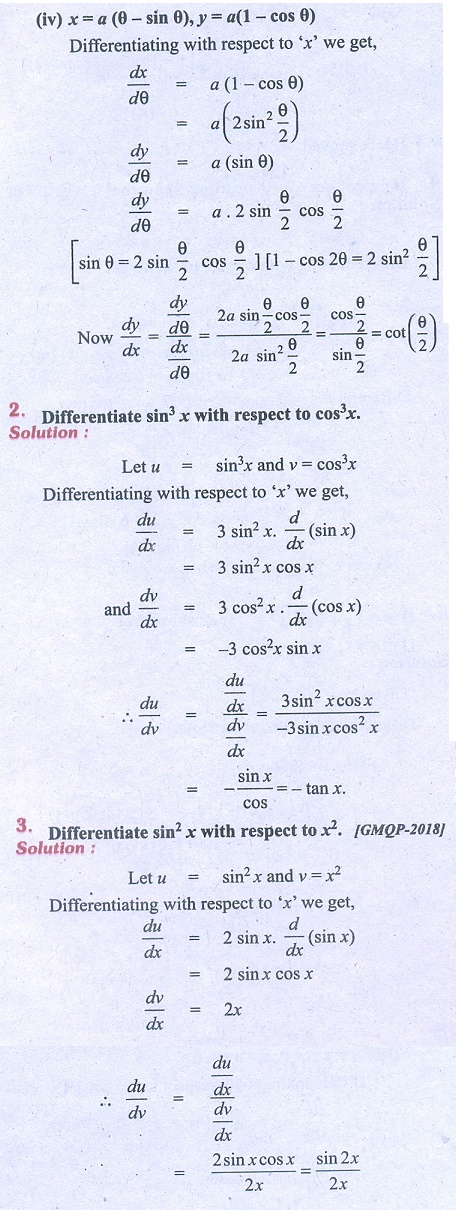Home | | Business Maths 11th std | Exercise 5.8: Differentiation of parametric functions

# Exercise 5.8: Differentiation of parametric functions

Business Mathematics and Statistics Book back answers and solution for Exercise questions - Mathematics: Differential Calculus: Differentiation techniques: Differentiation of parametric functions, Differentiation of a function with respect to another functionTags : Problem Questions with Answer, Solution | Differential Calculus | Mathematics , 11th Business Mathematics and Statistics(EMS) : Chapter 5 : Differential Calculus
Study Material, Lecturing Notes, Assignment, Reference, Wiki description explanation, brief detail
11th Business Mathematics and Statistics(EMS) : Chapter 5 : Differential Calculus : Exercise 5.8: Differentiation of parametric functions | Problem Questions with Answer, Solution | Differential Calculus | Mathematics